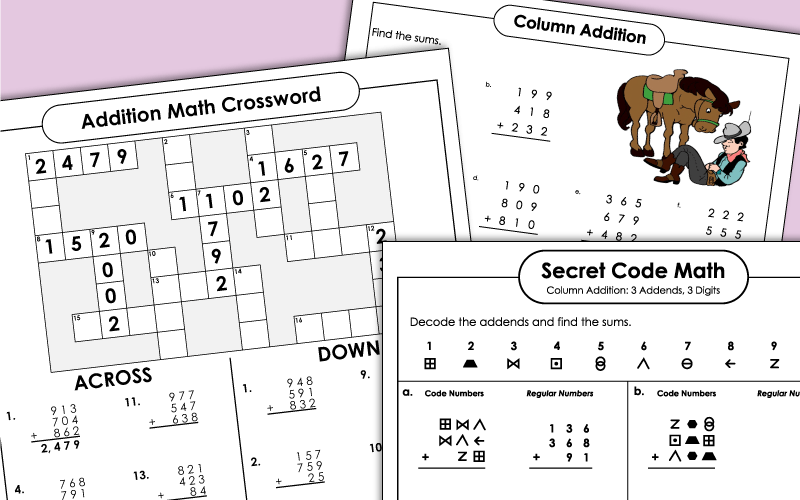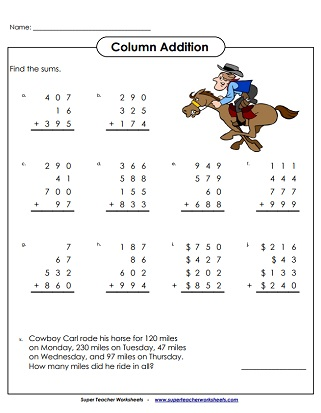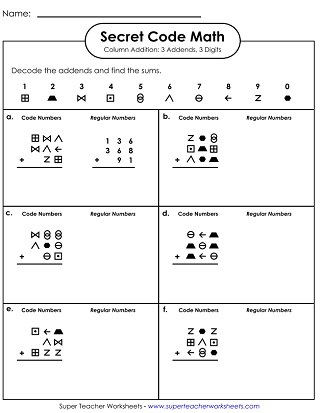Printable addition worksheets in which the problems have multiple addends (column addition).  These printable feature one, two, three, and four-digit numbers.## Two-Digit

Double-digit column addition problems with three or four addends; Includes one word problem
Look for mistakes in these completed addition problems. Explain the errors. Then solve.

## Three-Digit

What do boats eat in the morning for breakfast? To solve the riddle, students will need to complete column addition problems. Each one has three, 3-digit addends.
Three-digit column addition problems with three  or four addends; Includes one word problem
Add the three digit numbers and plug them into the math crossword puzzle.
Kids look through a grocery store ad paper and choose 3 foods they'd like to buy.  They cut out the pictures and prices, then glue them onto the worksheet.  Then they must add the prices of the 3 items together to find the sum.
Four word problems that require students to use column addition to solve; Includes space for students to show their work
More story problems in which students practice column adding
These problems were incorrectly solved. Students must explain the mistakes, then re-solve the problems correctly.
Here's a fun code math activity your kids will enjoy. First, students will use the key to decipher the graphical symbols in the addends. Then they solve the problems.

## Four-Digit

What do you call a skeleton that won't work? To find the answer to this riddle, students solve a series of 4-digit column addition problems.
These problems have four addends with 4 digits. The problems have already been completed, but there are mistakes. Students must explain the errors and solve the problems correctly.
In this secret code activity, students will use the cipher key to decode sets of three or four addends. Then they'll find the sum of each set.

Daily Word Problems

Print a new word problem for students to solve each day.My Account
Site Information